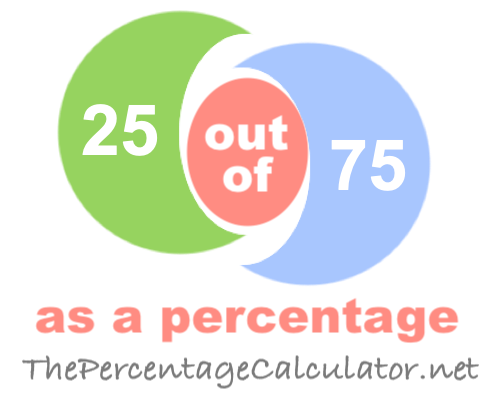What is 25 out of 75 as a percentage?When you ask "What is 25 out of 75?" you want to know what percent 25 is out of 75.

Here are step-by-step instructions showing you how we calculated 25 out of 75 as a percentage:

The first step is to divide 25 by 75 to get the answer in decimal form:

25 / 75 = 0.3333

Then, we multiplied the answer from the first step by one hundred to get the answer as a percentage:

0.3333 * 100 = 33.33%

We can prove that the answer is correct by taking 33.33 percent of 75 to get 25:

(75 x 33.33)/100 = 25

Note that our calculator rounds the answers up to two decimals if necessary. Once again, the answer is as follows:

33.33%

Out of as a Percentage Calculator
To solve another problem, please submit it below:

out of

What is 25 out of 76 as a percentage?
The solution to "What is 25 out of 75 as a percentage?" is not the only answer we have. Go here for the next solution on our list.

Copyright  |   Privacy Policy  |   Disclaimer  |   Contact